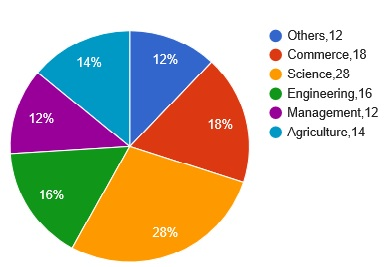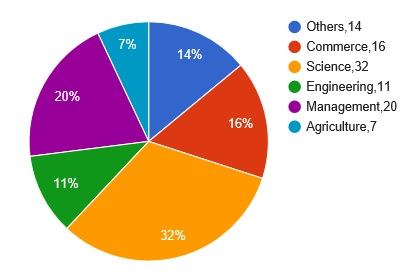### SBI Associate PO 2010 Question 147

Instructions

Study the following pie-chart carefully and answer the question given below:
Discipline wise Break up of Numbers of candidates appeared in Interview and Discipline Break up of Number of Candidates selected by an organisation
Discipline Wise Break up of Number of candidates appeared in Interview by the organisation
Total Number of candidates Appeared
In the Interview=25780 percentageDiscipline Wise Break up of Number of candidates selected after Interview by organisation
Total Number of Candidates selected After
Interview=7390 percentageQuestion 147

# The total number of candidates appeared in Interview from Management and other disciplines was what percent of number of candidates appeared from Engineering discipline ?

Solution

candidates appeared from management = (12/100)*25780 = 3093.6

from others = (12/100)*25780 = 3093.6

from eng. = (16/100)*25780 = 4124.8

reqd ans = (3093.6+3093.6)/4124.8 = 6187.2/4124.8 = 1.5

%age = 1.5*100 = 150

(shortcut)

total % from mgmt and others = 12 + 12 =24%

from eng = 16%

ans 24/16 = 1.5

=> 150%